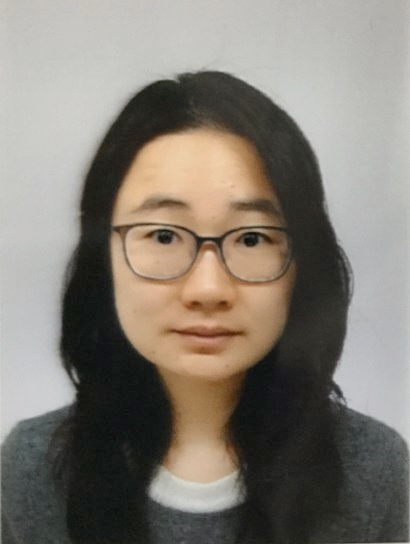### Comprehensive List of Researchers "Information Knowledge"

Department of Computer Science and Mathematical Informatics

Name
HU, Yannan
Group
Mathematical Modeling and Analysis Group
Title
Assistant Professor
Degree
Doctor of Information Science
Research Field
Combinatorial optimization#### Current Research

##### Heuristics for combinatorial optimization problems

[Outline]
Combinatorial optimization is one of the most active areas in operations research, computer science and discrete mathematics. It appears in various fields such as operations research, applied mathematics and theoretical computer science. Combinatorial optimization problems involve various applications, such as airline crew scheduling, VLSI design and network design. A variety of real-life problems can be abstracted as combinatorial optimization problems. Some of the classical problems in combinatorial optimization such as minimum spanning trees, shortest paths, network flows, matchings, etc., have polynomial time algorithms.

However, there are numerous combinatorial optimization problems such as the knapsack problem, traveling salesman problem, scheduling problem, packing problem, which are proved to be NP-hard. Under the widely believed conjecture that P¹NP, finding their exact solutions is prohibitively time consuming in the worst case. This negative aspect is not caused by missing more clever approaches to the problems but is indeed a general property of the NP-hard problems. Consequently, we have to look for other ways to deal with this situation. In particular, in many practical applications, it is not really necessary to find exact optimal solutions, and sufficiently good solutions would be enough. Hence, the approximation and heuristic algorithms that compute sufficiently good solutions in reasonable time become very important. The major topic of my research is to develop efficient heuristics for the combinatorial optimization problems.

[Topics]
Packing Problems:
Packing problems are classic combinatorial optimization problems and known to be NP-hard. Many packing problems are related to real-world applications and they have been studied for a long time from both theoretical and practical points of view. In a packing problem, it involves packing a set of objects, called items, into containers. The objective is to pack a single container as densely as possible, or pack all items into as few containers as possible. There are many variants of packing problems and my research focuses on the following problems.

• Two-dimensional Packing Problems:
• rectangle packing problem,
• rectilinear block strip packing problem,
• bitmap shape packing problem,
• irregular packing problem;
• Three-dimensional Packing Problem:
• three-dimensional strip packing problem;

Vehicle Routing Problem:
This research focuses on the multi-vehicle covering problem (m-CTP) that is a generalization of the set covering problem (SCP) and the vehicle routing problem (VRP). The SCP is a problem to determine an optimal combination of subsets that covers all given elements. The VRP is the problem of minimizing the total travel distance of a number of vehicles under various constraints, where every customer must be visited exactly once by a vehicle. Both of the SCP and VRP are representative combinatorial optimization problems and are known to be NP-hard.

Scheduling Problem:
This research considers the airline and bus crew scheduling problems that call for assigning crew members in order to cover all flights or bus trips with the minimum cost under a series of constraints. These problems frequently appear in real-world applications, such as those in bus and rail transit industry. In practical applications, it is difficult to create an efficient schedule satisfying all the constraints. We usually formulate such problems as set covering problems and apply column generation approach to generate candidate sets of schedules.

#### Career

• April, 2014 　Research Fellow, Japan Society for the Promotion of Science (JSPS)
• February, 2016 　Doctor of Information Science, Nagoya University
• March, 2016      Assistant Professor, Nagoya University